## 算法设计与分析A 复习总结2

2020/8/11 19:48:11 文章标签:

## 第三章 蛮力法

1.冒泡排序（BubbleSort）

• 算法思想：冒泡排序的原理（以递增序为例）是每次从头开始依次比较相邻的两个元素，如果后面一个元素比前一个要大，说明顺序不对，则将它们交换，本次循环完毕之后再次从头开始扫描，直到某次扫描中没有元素交换，说明每个元素都不比它后面的元素大，至此排序完成。

• 时间复杂度：①若初始状态是有序的，扫描一趟就可完成排序，此时的时间复杂度最优为O(n)
②若初始状态为逆序的，就需要n趟排序，总共需要比较和移动n(n-1)/2次，则时间复杂度最坏为O(n^2)
所以综上平均时间复杂度为O(n^2)

• 算法稳定性：冒泡排序就是把小的元素往前调或者把大的元素往后调。比较是相邻的两个元素比较，交换也发生在这两个元素之间。如果两个相等的元素相邻，那么根据我们的算法。它们之间没有发生交换；如果两个相等的元素没有相邻，那么即使通过前面的两两交换把两个相邻起来，这时候也不会交换，所以相同元素的前后顺序并没有改变，所以冒泡排序是一种稳定排序算法

• 算法实现

``````void BubbleSort(int a[], int n)
{
for(int i=0;i<n-1;i++)
for (int j = 0; j < n - i - 1; j++)
{
if (a[j] > a[j + 1])
{
int t = a[j]; a[j] = a[j + 1]; a[j + 1] = t;
}
}
}
``````

2.蛮力字符串匹配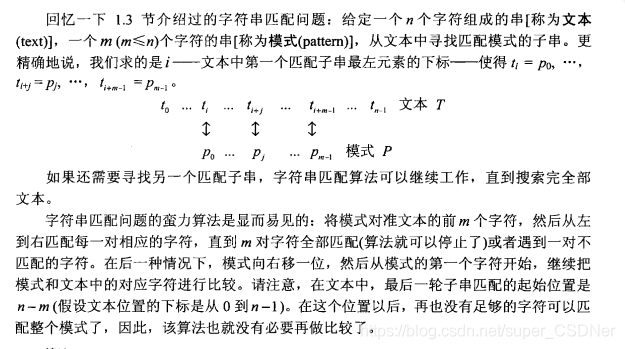``````int BruteForceStringMatch(int a[], int n,int b[],int m)
{
for (int i = 0; i <= n - m; i++)
{
int j = 0;
while (j < m&&b[j] == a[i + j])
{
j++;
if (j == m)return i;//匹配成功返回第一个匹配子串中的第一个字符的位置
}
}
return -1;
}
``````

3.凸包问题

• 凸集合：对于平面上的一个点集合，如果以集合中任意两点p和q为端点的线段都属于该集合，则这个集合是凸的
• 凸包：一个点集合S的凸包是包含S的最小凸集合(“最小”是指S的凸包一定是所有包含S的凸集合的子集)

4.深度优先查找(DFS)和广度优先查找(BFS)

(1)从某个顶点V出发，访问顶点并标记为已访问

(2)访问V的邻接点，如果没有访问过，访问该顶点并标记为已访问，然后再访问该顶点的邻接点，递归执行

(1)从某个顶点V出发，访问该顶点的所有邻接点V1，V2…VN

(2)从邻接点V1，V2…VN出发，再访问他们各自的所有邻接点

(3)重复上述步骤，直到所有的顶点都被访问过

## 第四章 减治法

1.插入排序(InsertionSort)：

• 算法思想：把待排序的记录按其关键码值的大小逐个插入到一个已经排好序的有序序列中，直到所有的记录插入完为止，得到一个新的有序序列。

• 时间复杂度：①若初始状态是有序的，扫描一趟就可完成排序，此时的时间复杂度最优为O(n)
②若初始状态为逆序的，就需要n趟排序，总共需要比较和移动n(n-1)/2次，则时间复杂度最坏为O(n^2)
所以综上平均时间复杂度为O(n^2)

• 算法稳定性稳定排序算法

• 算法实现

``````void InsertionSort(int a[], int n)
{
for (int i = 1; i < n; i++)
{
int t = a[i];
int j = i - 1;
while (j >= 0 && a[j] > t)
{
a[j + 1] = a[j];
j--;
}
a[j + 1] = t;
}
}
``````

2.拓扑排序

• 拓扑排序是指将一个有向无环图(Directed Acyclic Graph简称DAG)进行排序进而得到一个有序的线性序列。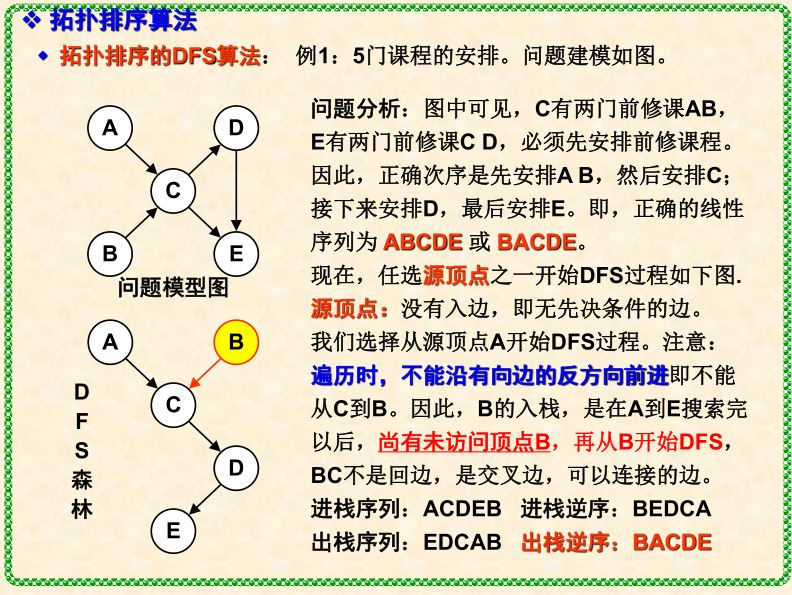## 第五章 分治法

1.合并排序

• 合并排序的基本思想：把待排序的n个元素分解成n组，也就是每组一个元素；之后对分好的组进行两两合并（无配对的则不操作），以此类推
• 时间复杂度：O(nlogn);
• 空间复杂度:O(n);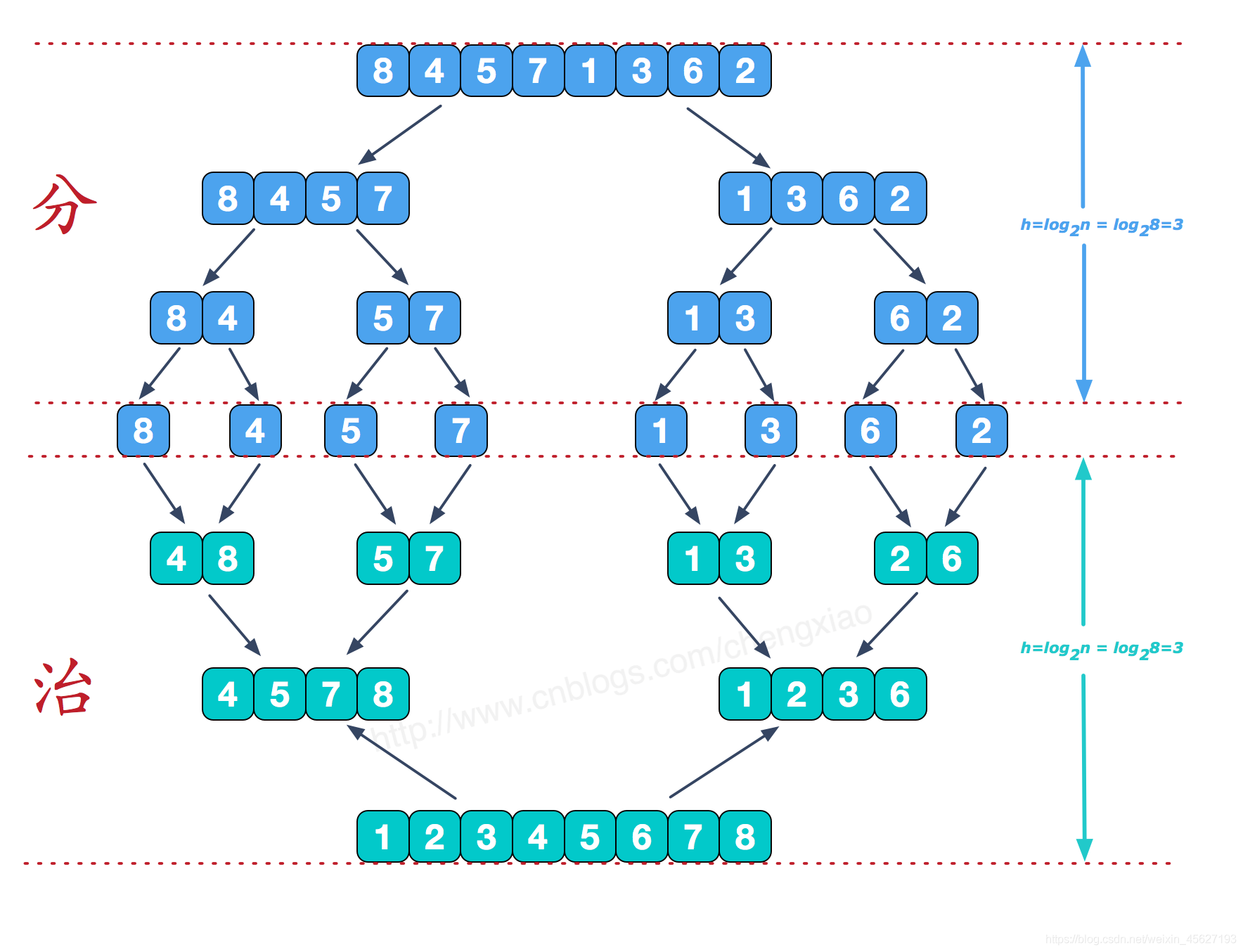``````#include<iostream>
using namespace std;
template<typename T>
void merge(T a[], int first, int mid, int last, T temp[])
{
int i = first,j=mid+1;
int m = mid,n=last,k=0;
while (i <= m && j <= n)
{
if (a[i] < a[j])temp[k++] = a[i++];
else temp[k++] = a[j++];
}
while (i <= m)
temp[k++] = a[i++];
while(j<=n)
temp[k++] = a[j++];
for (i = 0; i < k; i++)
a[i+first] = temp[i];
}
template<typename T>
void mergesort(T a[], int first, int last, T temp[])
{
if (first < last)
{
int mid = (first + last) / 2;
mergesort(a, first, mid, temp);
mergesort(a, mid + 1, last, temp);
merge(a, first, mid, last, temp);
}
}
void main()
{
int a = { 8,3,2,6,7,1,5,4 }, temp = { 0 };
mergesort(a, 0, 7, temp);
for (int i = 0; i < 8; i++)
cout << a[i] << " ";
cout << endl;
system("pause");
}
``````

2.快速排序

• 基本思想：在数组中选一个基准数（通常为数组第一个）； 将数组中小于基准数的数据移到基准数左边，大于基准数的移到右边；对于基准数左、右两边的数组，不断重复以上两个过程，直到每个子集只有一个元素，即为全部有序
• 时间复杂度：O(nlogn);
• 空间复杂度:O(nlogn);

``````void quick_sort(int a[], int begin, int end)
{
if (begin < end)
{
int i = begin, j = end,temp=a[begin];
while (i < j)
{
while (i<j&&a[j]>temp)
j--;
a[i] = a[j];
while (i < j&&a[i]<=temp)
i++;
a[j] = a[i];
}
a[i] = temp;
quick_sort(a, begin, i - 1);
quick_sort(a, i + 1, end);
}
}
``````

## 第六章 变治法

1.平衡二叉树(BST)：AVL树和2-3树

AVL树;

• 定义： ：AVL 树是一棵BST ，树中每个节点的 平衡因子 定义为该节点的左子树高度减右子树高度 。空树高度为0 。注意本讲义的树高定义—— 从根到叶的最长路径上的边数。定义根为0 层，那么树高等于最底层的层编号。AVL树的平衡因子为0，-1，+1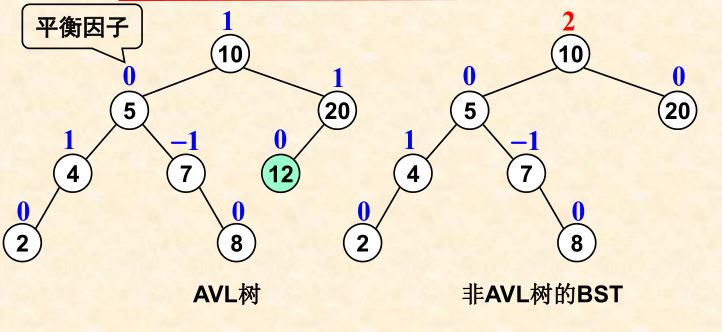2.2-3树：2节点和3节点

• 一个2节点只包含一个键K和两个子女，左子女作为一棵所有键都小于K的子树的根，而右子女作为一棵所有键都大于K和两个子女的子树的根。(换句话说，一个2节点和一棵二叉查找树的节点类型相同)
• 一个3节点包含两个有序的键K1,K2（K1<K2）并且有3个子女，最左边的子女为键值小于K1的子树的根，中间的子女作为键值位于K1和K2之间的子树的根，最右边的子女作为键值大于K2的子树的根
• 树中的所有叶子必须位于同一层，一棵2-3树是高度平衡的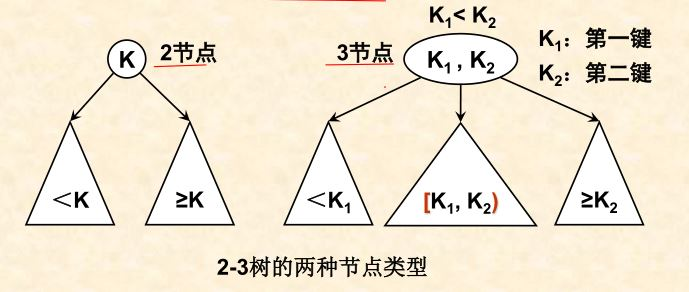3.

• 必须是完全二叉树：每一层都是满的，除了最后一层的最右边可能有缺位
• 父母优势：每个节点的键值都要大于或等于它子女的键

• 只存在一棵n个节点的完全二叉树，它的高度为 logn
• 堆的根总是包含了堆的最大元素
• 堆的一个节点以及该节点的子孙也是一个堆

## 第八章 动态规划(DP)

1.DP和分治法的区别：

• 分治法是把问题分成独立的子问题，各个子问题能独立解决
• DP的子问题之间是相关的，前面子问题的解决结果被后面的子问题使用
• DP适合于有重叠子问题和最优子结构性质的问题

2.动态规划的特点：

• 把原始问题划分为一系列子问题
• 求解每个子问题仅一次，并将其结果保存在一个表中，以后用到时到时直接存取，不重复计算，节省计算时间
• 自底向上地计算

3.最优二叉查找树

• 定义：给定n个互异的关键字组成的序列K=<k1,k2,…,kn>，且关键字有序（k1<k2<…<kn），我们想从这些关键字中构造一棵二叉查找树。对每个关键字ki，一次搜索搜索到的概率为pi。可能有一些搜索的值不在K内，因此还有n+1个“虚拟键”d0,d1,…,dn，他们代表不在K内的值。具体：d0代表所有小于k1的值，dn代表所有大于kn的值。而对于i = 1,2,…,n-1,虚拟键di代表所有位于ki和ki+1之间的值。对于每个虚拟键，一次搜索对应于di的概率为qi。要使得查找一个节点的期望代价（代价可以定义为：比如从根节点到目标节点的路径上节点数目）最小，就需要建立一棵最优二叉查找树。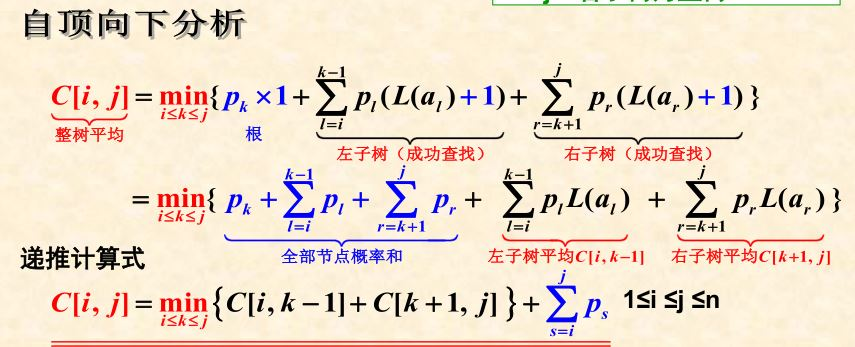建立一棵二叉查找树，如果是的上式最小，那么这棵二叉查找树就是最优二叉查找树。

4.Prime算法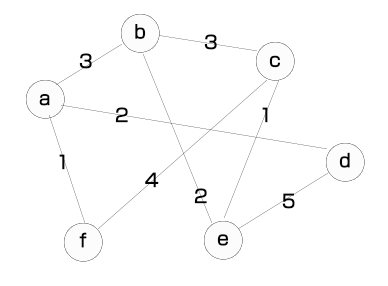① 顶点a发出的边包括<a,b>和<a,d>和<a,f>，其中权重最小的边为<a,f>，于是我们将边<a,f>加入到最小生成树中，此时最小生成树包括下图中的阴影边和灰色顶点。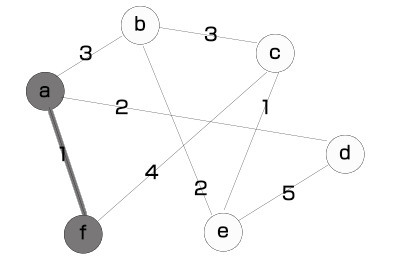② 接下来我们继续从当前最小生成树中的顶点发出的所有边中寻找权重最小的一条，即边<a,b>、<a,d>、<f,c>中的边<a,d>，于是我们将边<a,d>加入到树中，如下图所示。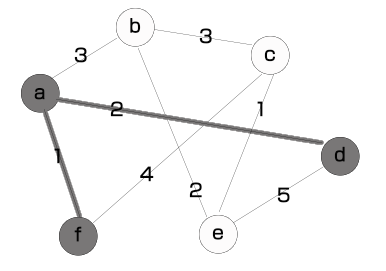③ 继续上述步骤，从顶点a、f、d发出的边中选出权重最小的一条，即边<a,b>，并将它加入树中，如下图所示。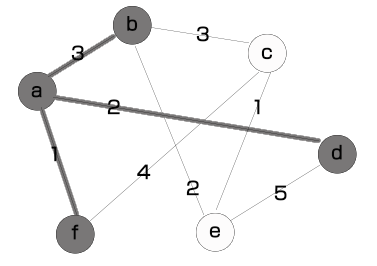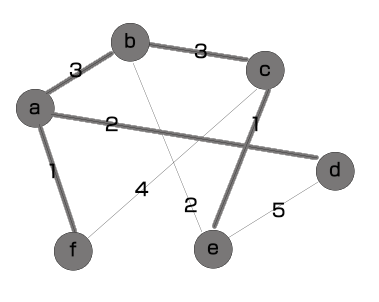暂无相关的数据...